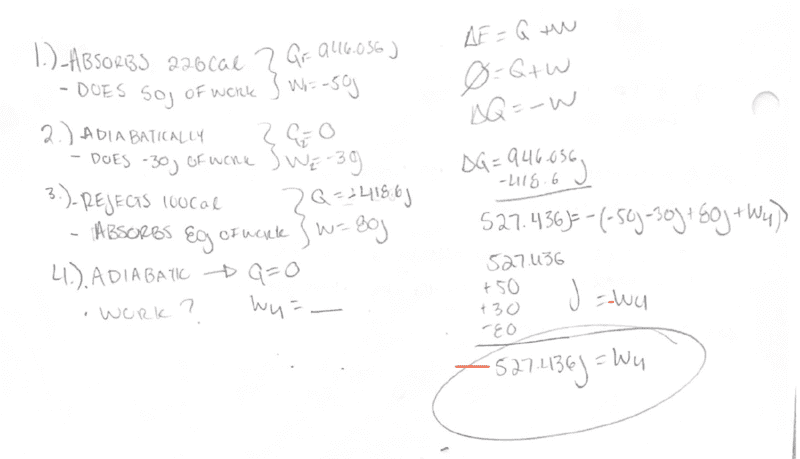# Evaluating a cycle [Thermodynamics]

guyvsdcsniper
Homework Statement:
Cycle. A system undergoes the following four- stage cyclic process:
In stage (1) the system absorbs 226 cal of heat and does 50 J of work; in
(2) the system adiabatically does 30 J of work; and in (3) the system rejects
100 cal of heat while the environment does 80 J of work on the system.
Stage (4) is also adiabatic. Is work done on or by the system in stage (4)?
How many joules of work?
Relevant Equations:
Q+W=0
Since this is a cycle, there should leave the system unchanged, hence Q+W = 0.

I wrote evaluated the heat and work done on/by the system at each cycle as shown in my work.

The first question ask is work done at the fourth stage. Since this a cycle and again the system she remain unchanged, and assuming the fourth stage is the last stage I can try to determine this by analyzing each cycle. The first stage absorbs 943.036J of heat. At the third stage, -418.6J of heat is expelled. That makes ΔQ = 527.436.

If the fourth stage is adiabatic, no heat can transfer at this stage. But since this is a cycle, the system must remain unchanged at the end. So that means there must be work done at the fourth stage in order to return back to the overall energy we started with.

We can say ΔQ=-W with W being each work done at each stage, -(W1+W2+W3+W4)

By algebra W4 = -527.436J.

So the system does work at this stage.

Is my approach correct?Homework Helper
Gold Member

•guyvsdcsniper
guyvsdcsniper
The end result should be negative right? I forgot to carry the negative in my original solution and had to add it in the red.

Homework Helper
Gold Member
The end result should be negative right? I forgot to carry the negative in my original solution and had to add it in the red.
You got a negative value for ##W_4##. But now you have to interpret this to decide if 527 J of work was done on or by the system during step 4.

guyvsdcsniper
You got a negative value for ##W_4##. But now you have to interpret this to decide if 527 J of work was done on or by the system during step 4.
It should be done by the system on step 4 correct?

Homework Helper
Gold Member
It should be done by the system on step 4 correct?
Yes, that's right. Can you give the reason for this?

guyvsdcsniper
Yes, that's right. Can you give the reason for this?
So if the fourth stage is adiabatic, this means that no heat can transfer at this stage. And this is also a cycle so the system must remain unchanged at the end. So Q+W=0.

Up until stage 4 all the work done on/by the system has canceled out and we only have a change of heat remaining. The heat was positve and when Q > 0 heat is absorbed by the system. Since W < 0, Work is done by the system in order to expell that internal energy/heat?

Is that correct?

Homework Helper
Gold Member
So if the fourth stage is adiabatic, this means that no heat can transfer at this stage. And this is also a cycle so the system must remain unchanged at the end. So Q+W=0.

Up until stage 4 all the work done on/by the system has canceled out and we only have a change of heat remaining. The heat was positve and when Q > 0 heat is absorbed by the system. Since W < 0, Work is done by the system in order to expell that internal energy/heat?

Is that correct?
Yes. Good.

As you stated in your first post, ##Q+W = 0## for any cycle. This assumes that ##W## is defined as work done on the system. Since you found ##W_4 =## -527 J, it means that -527 J was done on the system in step 4. This is equivalent to saying +527 J was done by the system in step 4.

You were probably already clear on this, but I just wanted to make sure. Signs can sometimes be tricky. You will find some books writing ##Q-W = 0## for a cycle because they define ##W## as work done by the system.

•vela and guyvsdcsniper
Homework Helper
Gold Member
I see now that you already dealt with the signs in this thread.